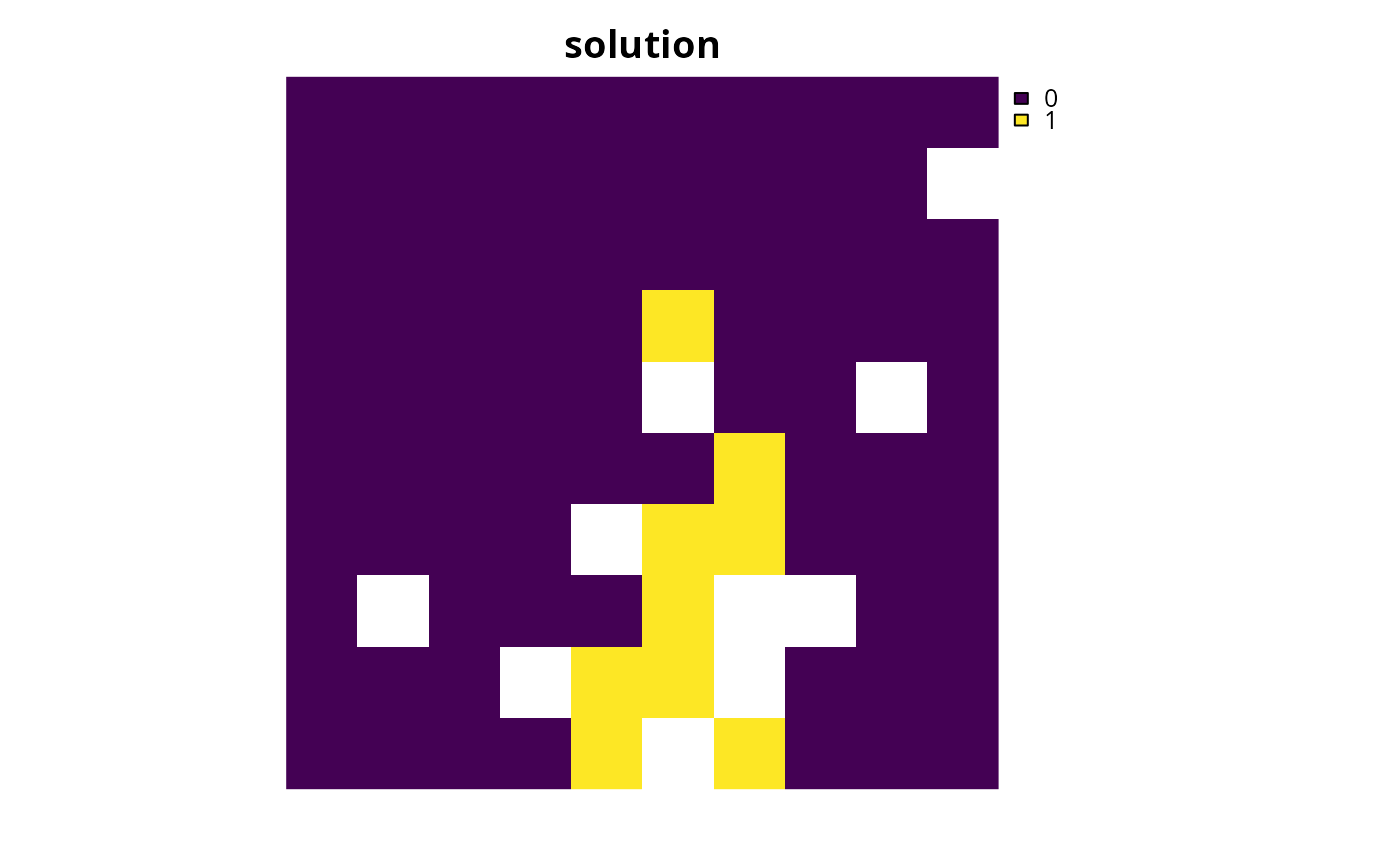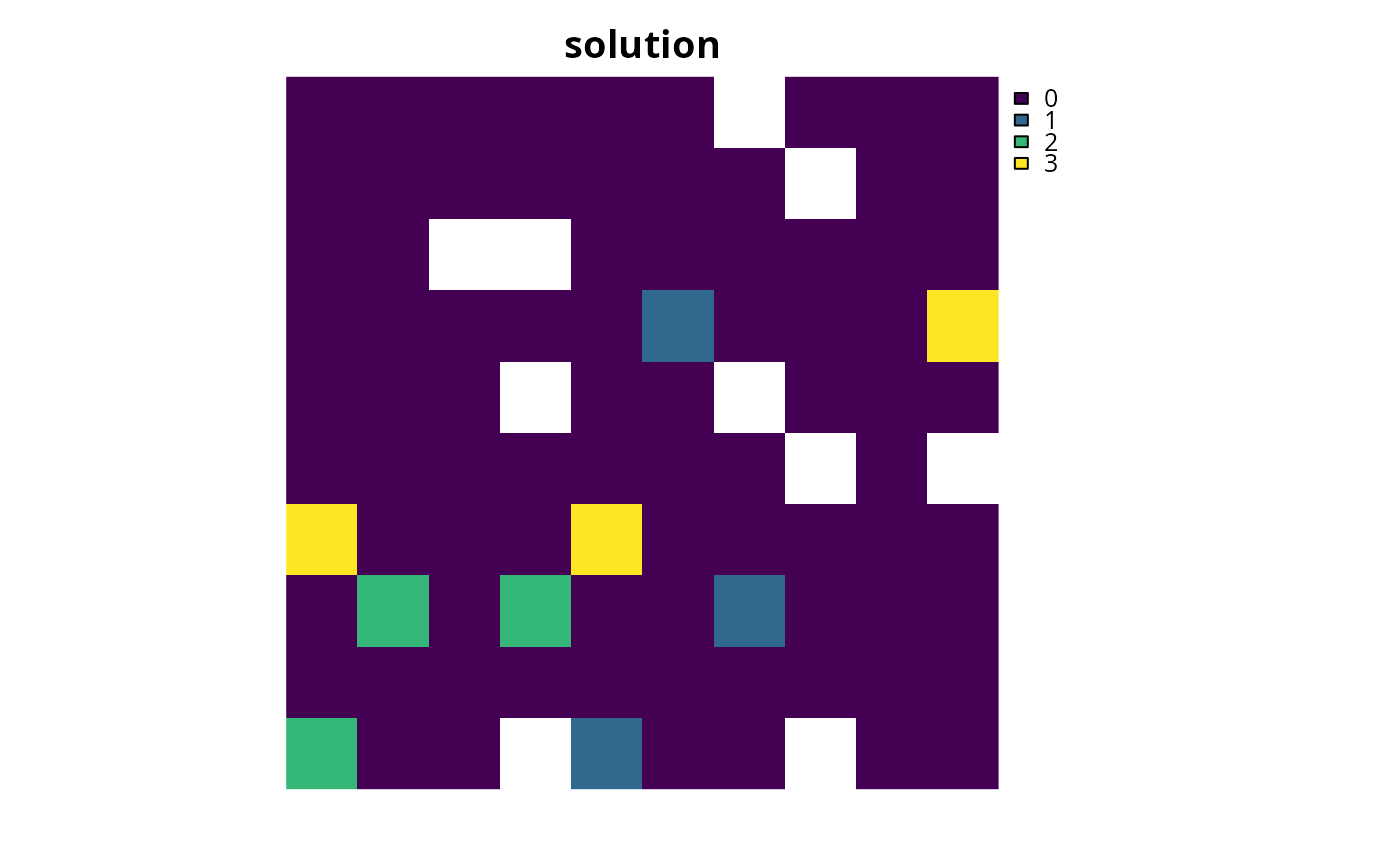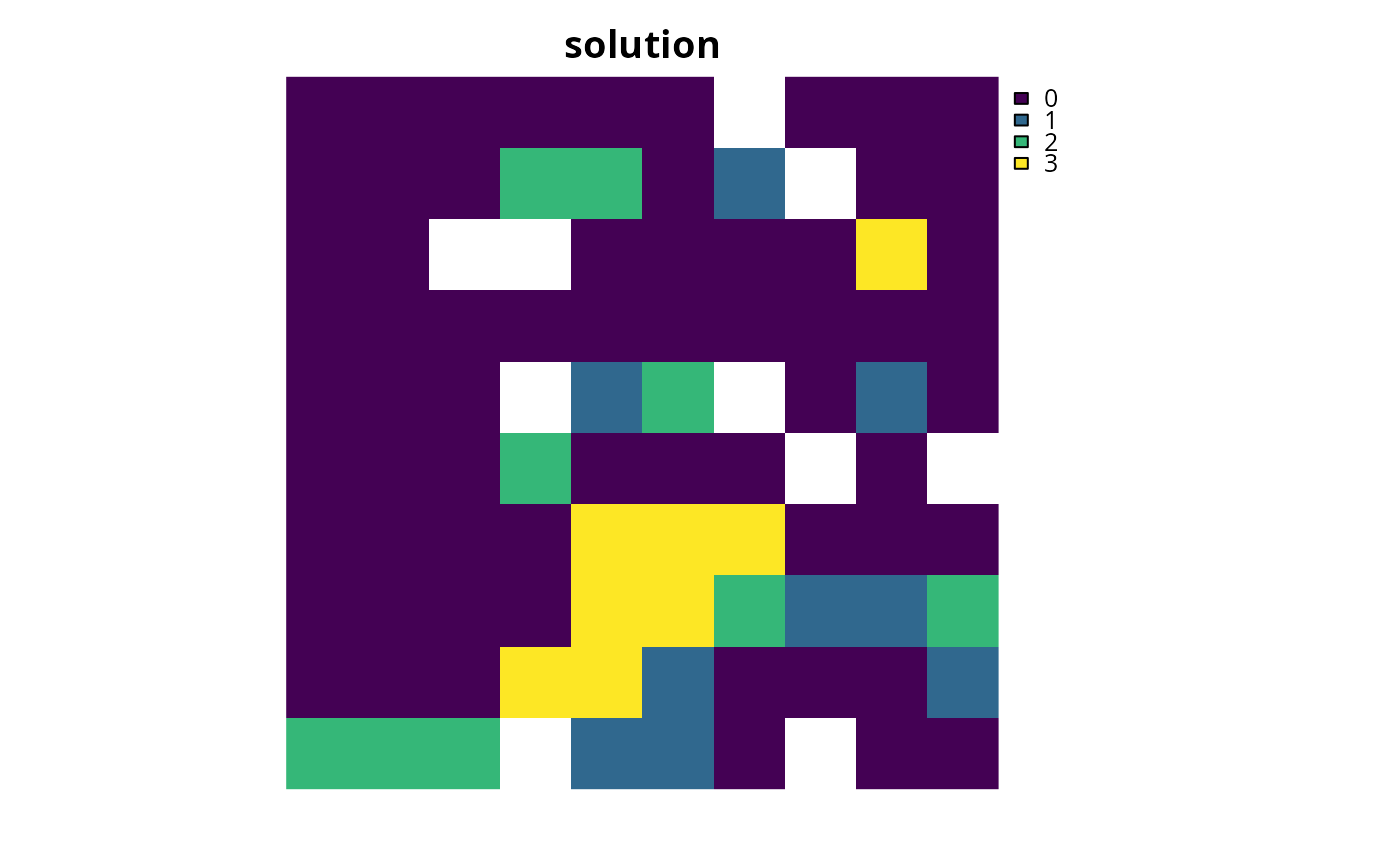Set the objective of a conservation planning problem to minimize the largest target shortfall while ensuring that the cost of the solution does not exceed a budget. Note that if the target shortfall for a single feature cannot be decreased beyond a certain point (e.g., because all remaining planning units occupied by that feature are too costly or are locked out), then solutions may only use a small proportion of the specified budget.

## Usage

add_min_largest_shortfall_objective(x, budget)

## Arguments

x

problem() object.

budget

numeric value specifying the maximum expenditure of the prioritization. For problems with multiple zones, the argument to budget can be (i) a single numeric value to specify a single budget for the entire solution or (ii) a numeric vector to specify a separate budget for each management zone.

## Value

An updated problem() object with the objective added to it.

## Details

The minimum largest shortfall objective aims to find the set of planning units that minimize the largest shortfall for any of the representation targets---that is, the fraction of each target that remains unmet---for as many features as possible while staying within a fixed budget. This objective is different from the minimum shortfall objective (add_min_shortfall_objective()) because this objective minimizes the largest (maximum) target shortfall, whereas the minimum shortfall objective minimizes the total (weighted sum) of the target shortfalls. Note that this objective function is not compatible with feature weights (add_feature_weights()).

## Mathematical formulation

This objective can be expressed mathematically for a set of planning units ($$I$$ indexed by $$i$$) and a set of features ($$J$$ indexed by $$j$$) as:

$$\mathit{Minimize} \space l \\ \mathit{subject \space to} \\ \sum_{i = 1}^{I} x_i r_{ij} + y_j \geq t_j \forall j \in J \\ l \geq \frac{y_j}{t_j} \forall j \in J \\ \sum_{i = 1}^{I} x_i c_i \leq B$$

Here, $$x_i$$ is the decisions variable (e.g., specifying whether planning unit $$i$$ has been selected (1) or not (0)), $$r_{ij}$$ is the amount of feature $$j$$ in planning unit $$i$$, and $$t_j$$ is the representation target for feature $$j$$. Additionally, $$y_j$$ denotes the target shortfall for the target $$t_j$$ for feature $$j$$, and $$l$$ denotes the largest target shortfall. Furthermore, $$B$$ is the budget allocated for the solution, $$c_i$$ is the cost of planning unit $$i$$. Note that $$y_j$$ and $$s$$ are continuous variables bounded between zero and infinity.

See objectives for an overview of all functions for adding objectives. Also, see targets for an overview of all functions for adding targets, and add_feature_weights() to specify weights for different features.

Other objectives: add_max_cover_objective(), add_max_features_objective(), add_max_phylo_div_objective(), add_max_phylo_end_objective(), add_max_utility_objective(), add_min_set_objective(), add_min_shortfall_objective()

## Examples

# \dontrun{
sim_pu_raster <- get_sim_pu_raster()
sim_features <- get_sim_features()
sim_zones_pu_raster <- get_sim_zones_pu_raster()
sim_zones_features <- get_sim_zones_features()

# create problem with minimum largest shortfall objective
p1 <-
problem(sim_pu_raster, sim_features) %>%

# solve problem
s1 <- solve(p1)

# plot solution
plot(s1, main = "solution", axes = FALSE)# create multi-zone problem with minimum largest shortfall objective,
# with 10% representation targets for each feature, and set
# a budget such that the total maximum expenditure in all zones
# cannot exceed 1800
p2 <-
problem(sim_zones_pu_raster, sim_zones_features) %>%
add_relative_targets(matrix(0.1, ncol = 3, nrow = 5)) %>%

# solve problem
s2 <- solve(p2)

# plot solution
plot(category_layer(s2), main = "solution", axes = FALSE)# create multi-zone problem with minimum largest shortfall objective,
# with 10% representation targets for each feature, and set
# separate budgets of 1800 for each management zone
p3 <-
problem(sim_zones_pu_raster, sim_zones_features) %>%